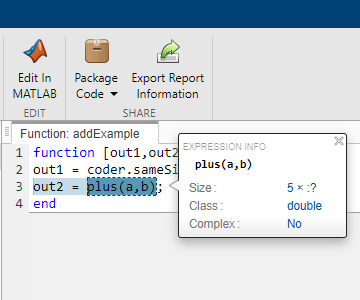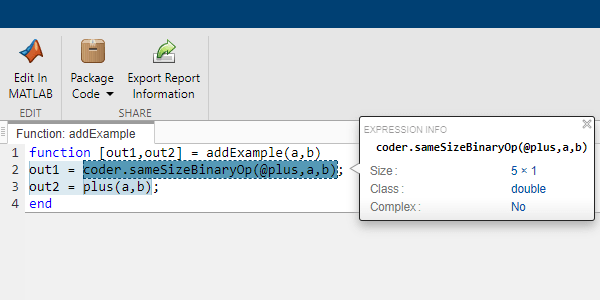coder.sameSizeBinaryOp

Apply element-wise binary operations without implicit expansion

Description

example

result = coder.sameSizeBinaryOp(func_handle,u,v) performs an error check that the operands are of the same size and applies the binary operation or function specified by the function handle func_handle on the operands u and v without implicitly expanding them. The operands must be of the same size because this function does not allow scalar expansion.

Use coder.sameSizeBinaryOp to disable implicit expansion for a specific binary operation or function. Disable implicit expansion to avoid automatic size change of output sizes, additional code generation, and performance variation. See Generate Code With Implicit Expansion Enabled, Optimize Implicit Expansion in Generated Code and Compatible Array Sizes for Basic Operations.

To disable implicit expansion for all binary operations and functions within a specific function in the generated code, call coder.noImplicitExpansionInFunction within that function.

Examples

collapse all

Use coder.sameSizeBinaryOp to apply binary operations and functions where implicit expansion is not required.

Using coder.sameSizeBinaryOp ensures that any variable-size operands of compatible sizes are not expanded automatically. The generated code does not include additional code to enable the automatic expansion of the operands.

In this example, the plus function applies the operation with implicit expansion. The coder.sameSizeBinaryOp function applies the operation without implicit expansion.

out1 = coder.sameSizeBinaryOp(@plus,a,b);
out2 = plus(a,b);
end

Define the input types.

a_type = coder.typeof(1,[5 1]);
b_type = coder.typeof(1,[5 inf]);

Generate code for the function addExample by using this command.

codegen addExample -args {a_type, b_type} -config:lib -report
Code generation successful: To view the report, open('codegen/lib/addExample/html/report.mldatx')

Compare Output Sizes

In the code generation report created in the previous step, place your cursor over the two operations.The size of the output from the plus operation is 5x:? whereas the size of the output of coder.sameSizeBinaryOp is 5x1.

The plus function implicitly expands its operands to the output size.

The coder.sameSizeBinaryOp function disables implicit expansion to prevent the automatic expansion of the operands.

Examine the Generated Code

/*
*
* MATLAB Coder version            : 5.3
* C/C++ source code generated on  : 23-Oct-2021 05:36:11
*/

/* Include Files */

/* Function Definitions */
/*
* Arguments    : const double a
*                const emxArray_real_T *b
*                double out1
*                emxArray_real_T *out2
* Return Type  : void
*/
void addExample(const double a, const emxArray_real_T *b, double out1,
emxArray_real_T *out2)
{
const double *b_data;
double *out2_data;
int i;
int i1;
int i2;
int loop_ub;
b_data = b->data;
for (i = 0; i < 5; i++) {
out1[i] = a[i] + b_data[i];
}
i = out2->size * out2->size;
out2->size = 5;
out2->size = b->size;
emxEnsureCapacity_real_T(out2, i);
out2_data = out2->data;
loop_ub = b->size;
for (i = 0; i < loop_ub; i++) {
for (i1 = 0; i1 < 5; i1++) {
i2 = i1 + 5 * i;
out2_data[i2] = a[i1] + b_data[i2];
}
}
}

/*
*
* [EOF]
*/

The code generated to calculate out1 by using coder.sameSizeBinaryOp is seen in the first for loop in the function addExample. The code generated to calculate out2 is seen below the first for loop. The generated code for out1 is much smaller, as compared to the code generated to calculate out2.

The code generated for the plus function needs additional code to expand its operands.

This example shows how to generate code for binary operations and functions without implicitly expanding its operands.

The coder.sameSizeBinaryOp function applies the required operation and bypasses additional code generation and change in output size associated with implicit expansion.

This example highlights the difference between the code generated for coder.sameSizeBinaryOp and the code generated for the minus function.

Subtracting Two Operands With Implicit Expansion

For this code snippet, the generated code implicitly expands the output.

type fooImpEx.m
function out = fooImpEx(a,b)
out = b - a;
end

Define the operand types.

a = coder.typeof(1,[2 1])
a =
coder.PrimitiveType
2×1 double

Edit Type Object

b = coder.typeof(1,[2 inf])
b =
coder.PrimitiveType
2×:inf double

Edit Type Object

Generate code for the function by running this command:

codegen fooImpEx -config:lib -args {a,b}
Code generation successful.

The code generated for the function fooImpEx is shown here.

type codegen/lib/fooImpEx/fooImpEx.c
/*
* File: fooImpEx.c
*
* MATLAB Coder version            : 5.3
* C/C++ source code generated on  : 23-Oct-2021 05:39:34
*/

/* Include Files */
#include "fooImpEx.h"
#include "fooImpEx_emxutil.h"
#include "fooImpEx_types.h"

/* Function Definitions */
/*
* Arguments    : const double a
*                const emxArray_real_T *b
*                emxArray_real_T *out
* Return Type  : void
*/
void fooImpEx(const double a, const emxArray_real_T *b, emxArray_real_T *out)
{
const double *b_data;
double *out_data;
int i;
int i1;
int loop_ub;
b_data = b->data;
i = out->size * out->size;
out->size = 2;
out->size = b->size;
emxEnsureCapacity_real_T(out, i);
out_data = out->data;
loop_ub = b->size;
for (i = 0; i < loop_ub; i++) {
out_data[2 * i] = b_data[2 * i] - a;
i1 = 2 * i + 1;
out_data[i1] = b_data[i1] - a;
}
}

/*
* File trailer for fooImpEx.c
*
* [EOF]
*/

The generated code includes code to automatically expand the size of compatible operands.

Subtracting Two Same-Size Operands Without Implicit Expansion

This code snippet uses coder.sameSizeBinaryOp to apply the operation without using implicit expansion.

type fooSameSize.m
function out = fooSameSize(a,b)
out = coder.sameSizeBinaryOp(@minus,b,a);
end

Generate code for the function by running this command:

codegen fooSameSize -config:lib -args {a,b}
Code generation successful.

The code generated for the function fooImpEx is shown here.

type codegen/lib/fooSameSize/fooSameSize.c
/*
* File: fooSameSize.c
*
* MATLAB Coder version            : 5.3
* C/C++ source code generated on  : 23-Oct-2021 05:39:38
*/

/* Include Files */
#include "fooSameSize.h"
#include "fooSameSize_types.h"

/* Function Definitions */
/*
* Arguments    : const double a
*                const emxArray_real_T *b
*                double out
* Return Type  : void
*/
void fooSameSize(const double a, const emxArray_real_T *b, double out)
{
const double *b_data;
b_data = b->data;
out = b_data - a;
out = b_data - a;
}

/*
* File trailer for fooSameSize.c
*
* [EOF]
*/

In this case, the variable out is fixed-size and the code generated for the operation applied by the coder.sameSizeBinaryOp function does not expand the operands. The generated function fooSameSize does not contain additional loops to increase the size of the operands.

Input Arguments

collapse all

Binary function to apply, specified as a function handle, func_handle must be a binary (two-input) element-wise function of the form C = func_handle(u,v) that accepts arrays u and v with the same-size. Apply these binary functions without implicit expansion by using coder.sameSizeBinaryOp:

FunctionSymbolDescription
plus+Plus
minus-Minus
times.*Array multiply
rdivide./Right array divide
ldivide.\Left array divide
power.^Array power
eq==Equal
ne~=Not equal
gt>Greater than
ge>=Greater than equal to
lt<Less than
le<=Less than equal to
and&Element-wise logical AND
or|Element-wise logical OR
xorN/ALogical exclusive OR
bitandN/ABit-wise AND
bitorN/ABit-wise OR
bitxorN/ABit-wise XOR
maxN/ABinary maximum
minN/ABinary minimum
modN/AModulus after division
remN/ARemainder after division
atan2dN/AFour-quadrant inverse tangent; result in degrees
hypotN/ASquare root of sum of squares

Example: result = coder.sameSizeBinaryOp(@plus, u, v);

Data Types: function_handle

Input array, specified as scalar, vector, matrix, or multidimensional array. Inputs u and v must be of the same sizes.

Data Types: single | double | int8 | int16 | int32 | int64 | uint8 | uint16 | uint32 | uint64 | logical | char | string | struct | table | cell | function_handle | categorical | datetime | duration | calendarDuration | fi
Complex Number Support: Yes

Input array, specified as scalar, vector, matrix, or multidimensional array. Inputs u and v must be of the same sizes.

Data Types: single | double | int8 | int16 | int32 | int64 | uint8 | uint16 | uint32 | uint64 | logical | char | string | struct | table | cell | function_handle | categorical | datetime | duration | calendarDuration | fi
Complex Number Support: Yes

Introduced in R2021b

MATLAB Coder Documentation